# 1670 Mystery Puzzle

### Today’s Puzzle:

The logic needed to solve this puzzle is a bit complicated. If you would like a hint, I’ll give one later in the post that will remove most of the mystery in the puzzle. Write the numbers 1 to 12 in both the first column and the top row so that those numbers and the given clues become a multiplication table.Logic Hint:
The 33 means that the 9 cannot be 3 × 3, so the 9 and the 54 must use both 9’s.
The 54 also must use a 6.
Thus the 36 near the bottom of the puzzle cannot be 9 × 4 or 6 × 6 and must be 3 × 12.
84 and that bottom 36 must use both 12’s, so the 48 must be 6 × 8 and not 12 × 4.
The rest of the puzzle should be rather straightforward.

### Factors of 1670:

• 1670 is a composite number.
• Prime factorization: 1670 = 2 × 5 × 167.
• 1670 has no exponents greater than 1 in its prime factorization, so √1670 cannot be simplified.
• The exponents in the prime factorization are 1, 1, and 1. Adding one to each exponent and multiplying we get (1 + 1)(1 + 1)(1 + 1) = 2 × 2 × 2 = 8. Therefore 1670 has exactly 8 factors.
• The factors of 1670 are outlined with their factor pair partners in the graphic below.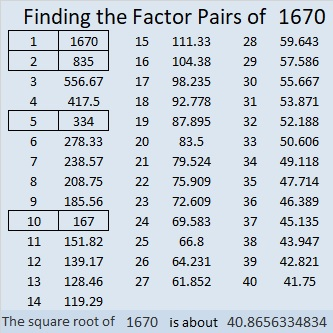### More About the Number 1670:

1670 is the hypotenuse of a Pythagorean triple:
1002-1336-1670, which is (3-4-5) times 334.

Although it is very much irrational, OEIS.org informs us that the first few digits of
1670^(1/6) is 3.44444624848…

### Today’s Puzzle:

Tomorrow is Independence Day in the United States. Happy Independence Day! Wherever you live, you can have a different kind of independence day, and it can happen any day of the year:

Are you dependent on a calculator, Siri, or someone or something else to give you any of the products or divisors in a multiplication table? Solving these Find the Factors puzzles can help you be more familiar with the table and declare your independence from those outside sources! Use logic to help you find its unique solution. Yes, mystery-level puzzles can be tricky, but I’ll give you a hint under the puzzle if you need it.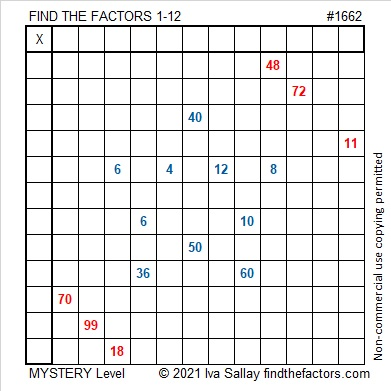The logic to get started: One column has 40, 50 and another column has 10, 60. There are only two numbers that can go at the top of either one of those columns: 5 and 10. We don’t know which column gets which number, however. But it is still enough to tell us that the other 5 and 10 must go in the first column with the 10 being a factor of 70 and the 5 being a factor of 50.

### Factors of 1662:

1662 is even, so it is divisible by 2.
1 + 2 = 3, so 1662 is divisible by 3. (Why wasn’t it necessary to include the 6’s in that calculation?)
Since 1662 is divisible by both 2 and 3, it is divisible by 6, too.

• 1662 is a composite number.
• Prime factorization: 1662 = 2 × 3 × 277.
• 1662 has no exponents greater than 1 in its prime factorization, so √1662 cannot be simplified.
• The exponents in the prime factorization are 1, 1, and 1. Adding one to each exponent and multiplying we get (1 + 1)(1 + 1)(1 + 1) = 2 × 2 × 2 = 8. Therefore 1662 has exactly 8 factors.
• The factors of 1662 are outlined with their factor pair partners in the graphic below.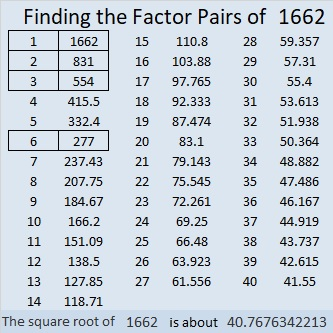### More About the Number 1662:

1662 is the hypotenuse of a Pythagorean triple:
690-1512-1662, which is 6 times (115-252-277).
Did you notice all the repeating digits in that triple?

# 1660 A 14×14 Mystery Puzzle

### Today’s Puzzle:

Adding a few more factors to the multiplication table really complicates this mystery-level puzzle. For example, will the common factor of 28 and 56 be 4, 7, or 14? If it were just a 10 × 10 or a 12 × 12 puzzle, answering that question would be easy. Not so with a 14 × 14 puzzle. Remember to use logic on every step while you find its unique solution.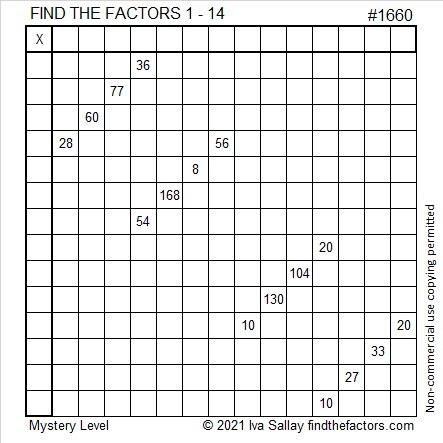You can print the puzzle or type the solution on this excel sheet: 10 Factors 1650-1660 with Taxman Scoring Calculator

### Factors of 1660:

• 1660 is a composite number.
• Prime factorization: 1660 = 2 × 2 × 5 × 83, which can be written 1660 = 2² × 5 × 83.
• 1660 has at least one exponent greater than 1 in its prime factorization so √1660 can be simplified. Taking the factor pair from the factor pair table below with the largest square number factor, we get √1660 = (√4)(√415) = 2√415.
• The exponents in the prime factorization are 2, 1, and 1. Adding one to each exponent and multiplying we get (2 + 1)(1 + 1)(1 + 1) = 3 × 2 × 2 = 12. Therefore 1660 has exactly 12 factors.
• The factors of 1660 are outlined with their factor pair partners in the graphic below.### More About the Number 1660:

1660 is the hypotenuse of a Pythagorean triple:
996-1328-1660, which is (3-4-5) times 332.

# 1659 Another Mystery

### Today’s Puzzle:

Both 20, 10, 30 and 12, 24, 36 have two possible common factors that will only put numbers from 1 to 10 in the first column and the top row of this mystery level puzzle. However, the puzzle has only one solution. Examine all the clues in the puzzle and think logically to determine what those common factors must be.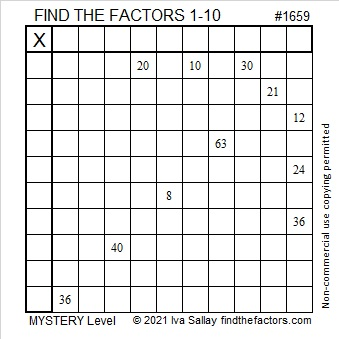### Factors of 1659:

• 1659 is a composite number.
• Prime factorization: 1659 = 3 × 7 × 79.
• 1659 has no exponents greater than 1 in its prime factorization, so √1659 cannot be simplified.
• The exponents in the prime factorization are 1, 1, and 1. Adding one to each exponent and multiplying we get (1 + 1)(1 + 1)(1 + 1) = 2 × 2 × 2 = 8. Therefore 1659 has exactly 8 factors.
• The factors of 1659 are outlined with their factor pair partners in the graphic below.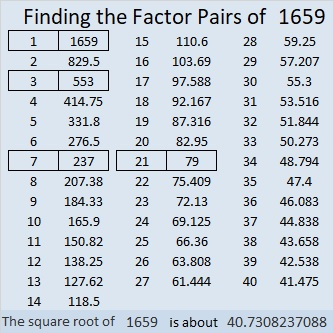### More About the Number 1659:

1659 is the difference of two squares in FOUR different ways:
830² – 829² = 1659,
278² – 275² = 1659,
122² – 115² = 1659, and
50² – 29² = 1659.

# 1658 Mystery Puzzle

### Today’s Puzzle:

What’s the mystery?
Will the common factor of 30 and 20 be 5 or 10?
Will the common factor of 36 and 18 be 6 or 9?
Will the common factor of 60 and 30 be 6 or 10? and
Will the common factor of 8 and 16 be 2, 4, or 8?

Don’t guess which common factors to use! Look at all the clues. They work together to help you logically arrive at the puzzle’s unique solution.### Factors of 1658:

• 1658 is a composite number.
• Prime factorization: 1658 = 2 × 829.
• 1658 has no exponents greater than 1 in its prime factorization, so √1658 cannot be simplified.
• The exponents in the prime factorization are 1 and 1. Adding one to each exponent and multiplying we get (1 + 1)(1 + 1) = 2 × 2 = 4. Therefore 1658 has exactly 4 factors.
• The factors of 1658 are outlined with their factor pair partners in the graphic below.### More About the Number 1658:

1658 is the sum of two squares:
37² + 17² = 1658.

1658 is the hypotenuse of a Pythagorean triple:
1080-1258-1658, which is 2 times (540-629-829),
and can also be calculated from 37² – 17², 2(37)(17), 37² + 17².

1658 is also a leg in the Pythagorean triple
calculated from 2(829), 829² – 1², 829² + 1².

# 1647 A Fun Mystery

### Today’s Puzzle:

I’ve never made a puzzle quite like this one before, and even though the logic needed is a little tricky, I found it quite enjoyable. Share it with a friend and see if you don’t have some great discussions such as if the common factor of 12 and 36 is 3, 4, 6, or 12.### Factors of 1647:

1 + 6 + 4 + 7 = 18, a multiple of 3 and 9, so 1647 is divisible by both 3 and 9.

• 1647 is a composite number.
• Prime factorization: 1647 = 3 × 3 × 3 × 61, which can be written 1647 = 3³ × 61.
• 1647 has at least one exponent greater than 1 in its prime factorization so √1647 can be simplified. Taking the factor pair from the factor pair table below with the largest square number factor, we get √1647 = (√9)(√183) = 3√183.
• The exponents in the prime factorization are 3 and 1. Adding one to each exponent and multiplying we get (3 + 1)(1 + 1) = 4 × 2 = 8. Therefore 1647 has exactly 8 factors.
• The factors of 1647 are outlined with their factor pair partners in the graphic below.### More About the Number 1647:

1647 is the hypotenuse of a Pythagorean triple:
297-1620-1647, which is 27 times (11-60-61).

1647 is a repdigit in base 13:
1647₁₀ = 999₁₃ because
9(13² + 13¹ + 13º) =
9(169 + 13 + 1) =
9(183) = 1647.

# 1646 Mystery Level

### Today’s Puzzle:

It’s a mystery if this puzzle is easy, difficult, or half easy and half difficult. The only way for you to know is to use logic to try solving it yourself!### Factors of 1646:

• 1646 is a composite number.
• Prime factorization: 1646 = 2 × 823.
• 1646 has no exponents greater than 1 in its prime factorization, so √1646 cannot be simplified.
• The exponents in the prime factorization are 1 and 1. Adding one to each exponent and multiplying we get (1 + 1)(1 + 1) = 2 × 2 = 4. Therefore 1646 has exactly 4 factors.
• The factors of 1646 are outlined with their factor pair partners in the graphic below.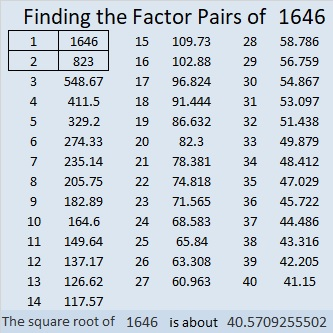### More About the Number 1646:

823 is part of the prime decade: 821, 823, 827, 829.
You can be sure that each of those primes doubled: 1642, 1646, 1654, and 1658 will have exactly four factors.

1646 is in only one Pythagorean triple:
1646-677328-677330, calculated from 2(823)(1), 823² – 1², 823² + 1².

# 1636 A Tricky Mystery Puzzle

### Today’s Puzzle:

It took me a while to figure out the logic of this puzzle so don’t get discouraged if you find it a bit tricky, too.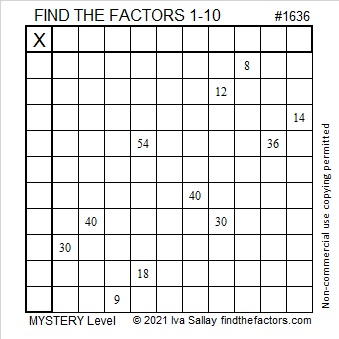If its logic eludes you, this short video I posted on Twitter should help.

### Factors of 1636:

• 1636 is a composite number.
• Prime factorization: 1636 = 2 × 2 × 409, which can be written 1636 = 2² × 409.
• 1636 has at least one exponent greater than 1 in its prime factorization so √1636 can be simplified. Taking the factor pair from the factor pair table below with the largest square number factor, we get √1636 = (√4)(√409) = 2√409.
• The exponents in the prime factorization are 2 and 1. Adding one to each exponent and multiplying we get (2 + 1)(1 + 1) = 3 × 2 = 6. Therefore 1636 has exactly 6 factors.
• The factors of 1636 are outlined with their factor pair partners in the graphic below.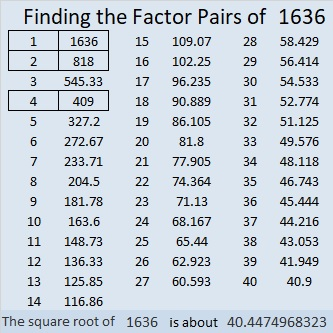### More about the Number 1636:

1636 is the sum of two squares:
40² + 6² = 1636.

1636 is the hypotenuse of a Pythagorean triple:
480-1564-1636, calculated from 2(40)(6), 40² – 6², 40² + 6².
It is also 4 times (120-391-409).

1636⁴ = 7,163,630,838,016. Thank you OEIS.org for that fun fact!

# 1635 The Logic to This Puzzle Is a Real Mystery

### Today’s Puzzle:

You can easily find a way to start this puzzle, but just a few factors later, it’s a mystery what to do next. Give it a shot, and see what I mean.Writing all the factor pairs for the clues often is helpful,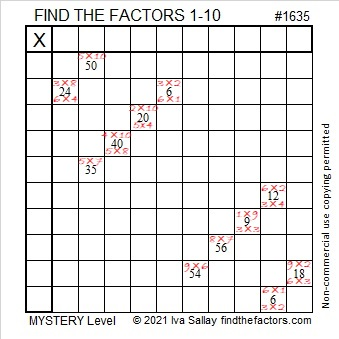but not as helpful as we might hope this time. Here’s a video explaining what to do to find a few more factors of this puzzle using logic:

### Factors of 1635:

• 1635 is a composite number.
• Prime factorization: 1635 = 3 × 5 × 109.
• 1635 has no exponents greater than 1 in its prime factorization, so √1635 cannot be simplified.
• The exponents in the prime factorization are 1, 1, and 1. Adding one to each exponent and multiplying we get (1 + 1)(1 + 1)(1 + 1) = 2 × 2 × 2 = 8. Therefore 1635 has exactly 8 factors.
• The factors of 1635 are outlined with their factor pair partners in the graphic below.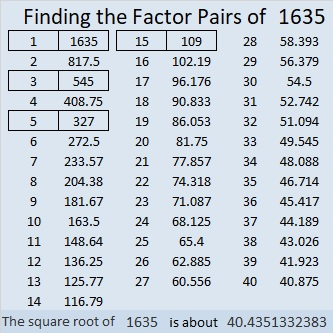### More about the Number 1635:

1635 is the hypotenuse of FOUR Pythagorean triples:
99-1632-1635, which is 3 times (33-544-545),
552-1539-1635, which is 3 times (184-513-545),
900-1365-1635, which is 15 times (60-91-109), and
981-1308-1635, which is (3-4-5) times 327.

OEIS.org informs us that there’s something special about the first nine decimals places of the fifth root of 1635.
Its fifth root is 4.392416875…
Can you figure out what is so special about that?

# 1616 Centering the Pendulum

### Today’s Puzzle:

Centering the Pendulum is Bill Davidson’s podcast about educators and how they inspire students to learn mathematics. All of his podcasts are wonderful and more than worth the 15 minutes or so needed to listen to each one.  I am quite honored that Find the Factors is the subject of his fourth podcast, and you can listen to it here.

To mark this occasion, I’ve made a mystery level puzzle that resembles a swinging pendulum that hopefully is centered! The puzzle might or might not be a little tricky, but just use logic every step of the way, and you should be fine.Using logic, write the numbers from 1 to 12 in both the first column and the top row so that those numbers and the given clues function like a multiplication table. Have fun!

### Factors of 1616:

• 1616 is a composite number.
• Prime factorization: 1616 = 2 × 2 × 2 × 2 × 101, which can be written 1616 = 2⁴ × 101
• 1616 has at least one exponent greater than 1 in its prime factorization so √1616 can be simplified. Taking the factor pair from the factor pair table below with the largest square number factor, we get √1616 = (√16)(√101) = 4√100.
• The exponents in the prime factorization are 4 and 1. Adding one to each exponent and multiplying we get (4 + 1)(1 + 1) = 5 × 2 = 10. Therefore 1616 has exactly 10 factors.
• The factors of 1616 are outlined with their factor pair partners in the graphic below.### More about the Number 1616:

1616 is the sum of two squares:
40² + 4² = 1616.

1616 is also the hypotenuse of a Pythagorean triple:
320-1584-1616, which is 16 times (20-99-101)
and can be calculated from 2(40)(4), 40² – 4², 40² + 4².

1616 is also the difference of two squares in three ways:
405² – 403² = 1616,
204² – 200² = 1616, and
105² – 97² = 1616.